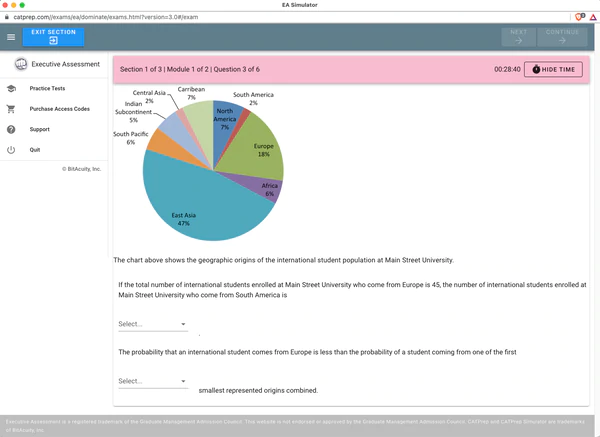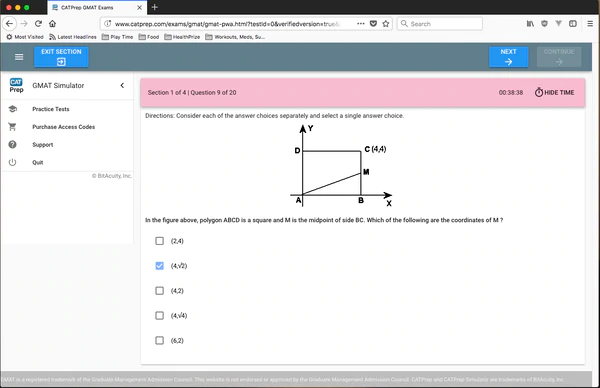# GRE Math Section

January 01, 0001 by CATPrep

# GRE Math Section

NOTE: The GRE Test is changing August 1st, 2011! Read about the new GRE test here.

The math or quantitative section has 2 different types of questions: regular multiple-choice questions, and inequalities. The inequalities always use the following format:

Column A Column B
value value
• you are given two columns with values, column A and column B.
• you are to decide which column has the greater value.
• if column A is larger, select option A
• if column B is larger, select option B
• if the two are equal, select option C
• if there isn't enough information, select option D.

The paper test will have similar questions all in the same section, but the computer test will mix and match questions that it asks you. make sure you read all the instructions for the question before answering.

Quantitative GRE questions will generally focus on subjects of geometry and algebra. Although a knowledge of trigonometry may be helpful for some problems that involve angles, circles, or triangles, it is not required. It is not necessary to know calculus or linear algebra to perform well on the quantitative section. It is important to note that unless it is explicitly stated in the question, you must assume that any images provided are not drawn to scale. It is therefore a bad idea to attempt to find an answer by graphical means. However, if you are an adept artist, you can attempt to draw your own figures to scale on the scrap paper, and attempt to find answers from those diagrams.

# Sample questions

```(not from actual tests)
```
Column A Column B
1 0
• if column A is larger, select option A

Column A Column B
-10 9
• if column B is larger, select option B

Column A Column B
1+1 2
• if the two are equal, select option C

Column A Column B
12 x+2
• if there isn't enough information, select option D.

# Applying algebra

A > B

• if column A is larger, select option A

A < B

• if column B is larger, select option B

A = B

• if the two are equal, select option C

A ??? B

• if there isn't enough information, select option D.

#### Latest PostsMay 09, 2022
###### CATPrep Pricing UpdateApril 04, 2022
###### CATPrep.com gets a makeoverMarch 14, 2022
###### Announcing Nine Executive Assessment Practice TestsJanuary 14, 2022
October 14, 2020
###### GRE test score tuning
September 24, 2020